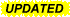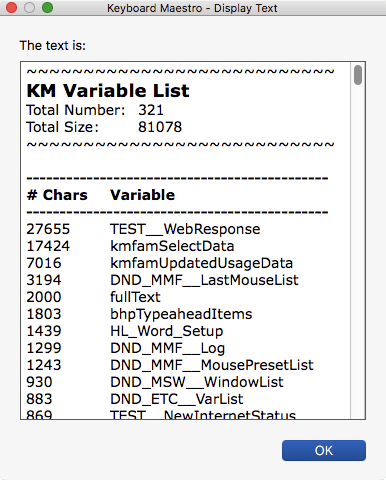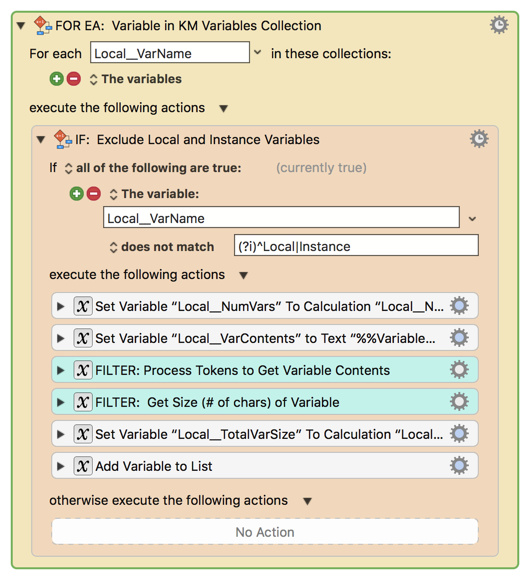# Get List of KM Variables and Size @KM @Tool

### MACRO:   Get List of KM Variables and Size @KM@Tool

~~~ VER: 1.1    2018-07-04 ~~~2018-07-04 22:14 GMT-5

• Added IF/THEN Action to exclude Local and Instance Variables from list.

## Get List of KM Variables and Size @KM @Tool.kmmacros (13 KB)Note: This Macro was uploaded in a DISABLED state. You must enable before it can be triggered.

### Example Output### ReleaseNotes

Author.@JMichaelTX

PURPOSE:

• Get List of KM Variables with Their Size

REQUIRES:

1. KM 8.2+
.
2. macOS 10.11.6 (El Capitan)
• KM 8 Requires Yosemite or later, so this macro will probably run on Yosemite, but I make no guarantees.How To Use

1. Open the KM Editor App
2. Trigger this macro.

MACRO SETUP

• Carefully review the Release Notes and the Macro Actions
• Make sure you understand what the Macro will do.
• You are responsible for running the Macro, not me. ??
.
1. Assign a Trigger to this maro..
2. Move this macro to a Macro Group that is only Active when you need this Macro.
3. ENABLE this Macro.

TAGS: @KM @Tool @Variables

• While I have given this limited testing, and to the best of my knowledge will do no harm, I cannot guarantee it.
• If you have any doubts or questions:
• Turn on the KM Debugger from the KM Status Menu, and step through the macro, making sure you understand what it is doing with each Action.4 Likes

@JMichaelTX I suggest to add one more action to filter meaningless local variables:

and a script action approach to separating out any locals might look something like:

``````(() => {
'use strict';

const main = () => {
const refVars = Application('Keyboard Maestro Engine').variables;

return unlines(
map(grp => unlines(
map(kn => `\${fst(kn)} :: \${snd(kn)}`,
grp
)
),

// Separated listing of any 'local'-prefixed variables,
// just in case this is embedded in a context which is
// creating temporary locals.
groupBy(
on(eq, x => fst(x).startsWith('local')),
sortBy(
comparing(fst),
zip(
refVars.name(),
map(length, refVars.value())
)
)
)
)
);
};

// GENERIC FUNCTIONS --------------------------------------

// https://github.com/RobTrew/prelude-jxa

// Tuple (,) :: a -> b -> (a, b)
const Tuple = (a, b) => ({
type: 'Tuple',
'0': a,
'1': b,
length: 2
});

// comparing :: (a -> b) -> (a -> a -> Ordering)
const comparing = f =>
(x, y) => {
const
a = f(x),
b = f(y);
return a < b ? -1 : (a > b ? 1 : 0);
};

// eq (==) :: Eq a => a -> a -> Bool
const eq = (a, b) => {
const t = typeof a;
return t !== typeof b ? (
false
) : t !== 'object' ? (
a === b
) : (() => {
const aks = Object.keys(a);
return aks.length !== Object.keys(b).length ? (
false
) : aks.every(k => eq(a[k], b[k]));
})();
};

// fst :: (a, b) -> a
const fst = tpl => tpl;

// Typical usage: groupBy(on(eq, f), xs)
// groupBy :: (a -> a -> Bool) -> [a] -> [[a]]
const groupBy = (f, xs) => {
const dct = xs.slice(1)
.reduce((a, x) => {
const h = a.active.length > 0 ? a.active : undefined;
return h !== undefined && f(h, x) ? {
active: a.active.concat([x]),
sofar: a.sofar
} : {
active: [x],
sofar: a.sofar.concat([a.active])
};
}, {
active: xs.length > 0 ? [xs] : [],
sofar: []
});
return dct.sofar.concat(dct.active.length > 0 ? [dct.active] : []);
};

// length :: [a] -> Int
const length = xs => xs.length;

// map :: (a -> b) -> [a] -> [b]
const map = (f, xs) => xs.map(f);

// e.g. sortBy(on(compare,length), xs)
// on :: (b -> b -> c) -> (a -> b) -> a -> a -> c
const on = (f, g) => (a, b) => f(g(a), g(b));

// snd :: (a, b) -> b
const snd = tpl => tpl;

// sortBy :: (a -> a -> Ordering) -> [a] -> [a]
const sortBy = (f, xs) =>
xs.slice()
.sort(f);

// unlines :: [String] -> String
const unlines = xs => xs.join('\n');

// zip :: [a] -> [b] -> [(a, b)]
const zip = (xs, ys) =>
xs.slice(0, Math.min(xs.length, ys.length))
.map((x, i) => Tuple(x, ys[i]));

// MAIN ----
return main();
})();

``````

Or (in Applescript):

Listing the largest Keyboard Maestro variables first, with a secondary sort by name:

``````use AppleScript version "2.4"
use framework "Foundation"

on run
tell application "Keyboard Maestro Engine"
tell variables
set ks to name
set vs to value
end tell
end tell

-- LARGEST KM VARIABLES LISTED FIRST
-- (secondary sort by name – case-insensitive)

unlines(map(reportLine, ¬
sortOn({{snd, false}, {lowerCaseName, true}}, ¬
zip(ks, map(|length|, vs)))))
end run

-- lowerCaseName :: (String, Int) -> String
on lowerCaseName(tpl)
toLower(fst(tpl))
end lowerCaseName

-- reportLine :: (String, Int) -> String
on reportLine(kn)
fst(kn) & " :: " & snd(kn) as text
end reportLine

-- GENERIC ----------------------------------------------

-- https://github.com/RobTrew/prelude-applescript

-- Tuple (,) :: a -> b -> (a, b)
on Tuple(a, b)
{type:"Tuple", |1|:a, |2|:b, length:2}
end Tuple

-- concatMap :: (a -> [b]) -> [a] -> [b]
on concatMap(f, xs)
tell mReturn(f)
set lng to length of xs
set acc to {}
repeat with i from 1 to lng
set acc to acc & |λ|(item i of xs, i, xs)
end repeat
end tell
return acc
end concatMap

-- flatten :: NestedList a -> [a]
on flatten(t)
if list is class of t then
concatMap(my flatten, t)
else
t
end if
end flatten

-- foldr :: (a -> b -> b) -> b -> [a] -> b
on foldr(f, startValue, xs)
tell mReturn(f)
set v to startValue
set lng to length of xs
repeat with i from lng to 1 by -1
set v to |λ|(item i of xs, v, i, xs)
end repeat
return v
end tell
end foldr

-- fst :: (a, b) -> a
on fst(tpl)
if class of tpl is record then
|1| of tpl
else
item 1 of tpl
end if
end fst

-- length :: [a] -> Int
on |length|(xs)
length of xs
end |length|

-- Lift 2nd class handler function into 1st class script wrapper
-- mReturn :: First-class m => (a -> b) -> m (a -> b)
on mReturn(f)
if class of f is script then
f
else
script
property |λ| : f
end script
end if
end mReturn

-- map :: (a -> b) -> [a] -> [b]
on map(f, xs)
tell mReturn(f)
set lng to length of xs
set lst to {}
repeat with i from 1 to lng
set end of lst to |λ|(item i of xs, i, xs)
end repeat
return lst
end tell
end map

-- min :: Ord a => a -> a -> a
on min(x, y)
if y < x then
y
else
x
end if
end min

-- Sort a list by comparing the results of a key function applied to each
-- element. sortOn f is equivalent to sortBy(comparing(f), xs), but has the
-- performance advantage of only evaluating f once for each element in
-- the input list. This is called the decorate-sort-undecorate paradigm,
-- or Schwartzian transform.
-- Elements are arranged from from lowest to highest.

-- In this Applescript implementation, f can optionally be [(a -> b)]
-- or [((a -> b), Bool)]) to specify a compound sort order

--    xs:  List of items to be sorted.
--          (The items can be records, lists, or simple values).
--
--    f:    A single (a -> b) function (Applescript handler),
--          or a list of such functions.
--          if the argument is a list, any function can
--          optionally be followed by a bool.
--          (False -> descending sort)
--
--          (Subgrouping in the list is optional and ignored)
--          Each function (Item -> Value) in the list should
--          take an item (of the type contained by xs)
--          as its input and return a simple orderable value
--          (Number, String, or Date).
--
--          The sequence of key functions and optional
--          direction bools defines primary to N-ary sort keys.
-- sortOn :: Ord b => (a -> b) -> [a] -> [a]
-- sortOn :: Ord b => [((a -> b), Bool)]  -> [a] -> [a]
on sortOn(f, xs)
script keyBool
on |λ|(x, a)
if class of x is boolean then
{asc:x, fbs:fbs of a}
else
{asc:true, fbs:({Tuple(x, asc of a)} & fbs of a)}
end if
end |λ|
end script
set {fs, bs} to {|1|, |2|} of unzip(fbs of foldr(keyBool, ¬
{asc:true, fbs:{}}, flatten({f})))

set intKeys to length of fs
set ca to current application
script dec
property gs : map(my mReturn, fs)
on |λ|(x)
set nsDct to (ca's NSMutableDictionary's ¬
dictionaryWithDictionary:{val:x})
repeat with i from 1 to intKeys
(nsDct's setValue:((item i of gs)'s |λ|(x)) ¬
forKey:(character id (96 + i)))
end repeat
nsDct as record
end |λ|
end script

script descrip
on |λ|(bool, i)
ca's NSSortDescriptor's ¬
sortDescriptorWithKey:(character id (96 + i)) ¬
ascending:bool
end |λ|
end script

script undec
on |λ|(x)
val of x
end |λ|
end script

map(undec, ((ca's NSArray's arrayWithArray:map(dec, xs))'s ¬
sortedArrayUsingDescriptors:map(descrip, bs)) as list)
end sortOn

-- snd :: (a, b) -> b
on snd(tpl)
if class of tpl is record then
|2| of tpl
else
item 2 of tpl
end if
end snd

-- toLower :: String -> String
on toLower(str)
set ca to current application
((ca's NSString's stringWithString:(str))'s ¬
lowercaseStringWithLocale:(ca's NSLocale's currentLocale())) as text
end toLower

-- unlines :: [String] -> String
on unlines(xs)
set {dlm, my text item delimiters} to ¬
{my text item delimiters, linefeed}
set str to xs as text
set my text item delimiters to dlm
str
end unlines

-- unzip :: [(a,b)] -> ([a],[b])
on unzip(xys)
set xs to {}
set ys to {}
repeat with xy in xys
set end of xs to |1| of xy
set end of ys to |2| of xy
end repeat
return Tuple(xs, ys)
end unzip

-- zip :: [a] -> [b] -> [(a, b)]
on zip(xs, ys)
set lng to min(length of xs, length of ys)
set lst to {}
repeat with i from 1 to lng
set end of lst to Tuple(item i of xs, item i of ys)
end repeat
return lst
end zip
``````
1 Like

Good idea. We need to exclude Instance variables as well.
So I put the entire block in the IF/THEN Action:I have updated the macro in my OP with this change.